# SQLite Arithmetic Operators

## Introduction

Arithmetic operators can perform arithmetical operations on numeric operands. There are four type of arithmetic operators: addition(+), subtraction(-), multiplication(*) and division(/).

Syntax:

``` SELECT <Expression>[arithmetic operator]<expression>...
FROM [table_name]
WHERE [expression];```
Parameter Description
Expression Expression made up of a single constant, variable, scalar function, or column name and can also be the pieces of an SQLite query that compare values against other values or perform arithmetic calculations.
arithmetic operator Plus(+), minus(-), multiply(*), and divide(/).
table_name Name of the table.

Example:

This is a simple example of using SQLite arithmetic operators :

Sample Output:

```SELECT 10+12-5*4/2;
10+12-5*4/2
-----------
12```

SQLite plus (+) operator

The SQLite plus (+) operator is used to add two or more expressions or numbers.

Example:

Sample table : customer

To get data of 'cust_name', 'opening_amt', 'receive_amt',  ('opening_amt' + 'receive_amt') from the 'customer' table with following condition -

1. sum of 'opening_amt' and  'receive_amt' is greater than 16000,

the following SQLite statement can be used:

``````SELECT cust_name, opening_amt, receive_amt,
FROM customer
WHERE (opening_amt + receive_amt) > 16000;
```
```

Relational Algebra Expression: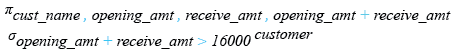Relational Algebra Tree: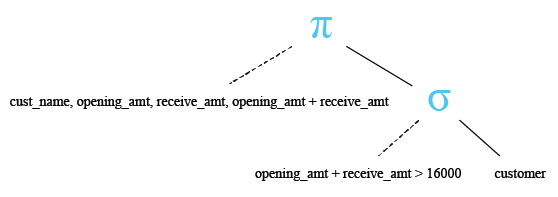Here is the result.

Sample Output:

```CUST_NAME   OPENING_AMT  RECEIVE_AMT  (opening_amt + receive_amt)
----------  -----------  -----------  ---------------------------
Sasikant    7000         11000        18000
Ramanathan  7000         11000        18000
Avinash     7000         11000        18000
Shilton     10000        7000         17000
Rangarappa  8000         11000        19000
Venkatpati  8000         11000        19000
Sundariya   7000         11000        18000
```

SQLite minus (-) operator

The SQLite minus (-) operator is used to subtracting one expression or number from another expression or number.

Example:>

To get data of 'cust_name', 'opening_amount', 'payment_amount' and 'oustanding_amount' from the 'customer' table with following condition -

1. 'outstanding_amt' - 'payment_amt' is equal to the  'receive_amt',

the following SQLite statement can be used :

``````SELECT cust_name, opening_amt, outstanding_amt
FROM customer
WHERE (outstanding_amt - payment_amt) = receive_amt;
```
```

Relational Algebra Expression: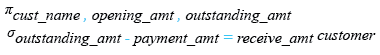Relational Algebra Tree: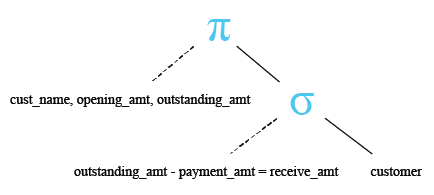Here is the result.

Sample Output:

```CUST_NAME   OPENING_AMT  OUTSTANDING_AMT
----------  -----------  ---------------
Stuart      6000         11000
```

SQLite multiply ( * ) operator

The SQLite multiply ( * ) operator is used to multiplying two or more expressions or numbers.

Example:

Sample table: agents

To get data of 'agent_code', 'agent_name', 'working_area' and  ('commission'*2) from the 'agents' table with following condition -

1. two times of the default 'commission' is greater than  0.25,

the following SQLite statement can be used :

``````SELECT agent_name, agent_name, working_area, (commission*2)
FROM agents
WHERE (commission*2) > 0.25;
```
```

Relational Algebra Expression: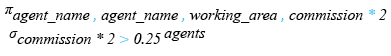Relational Algebra Tree: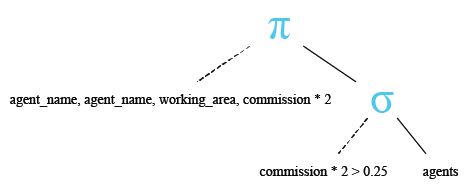Here is the result.

```AGENT_NAME  AGENT_NAME  WORKING_AREA  (commission*2)
----------  ----------  ------------  --------------
Ramasundar  Ramasundar  Bangalore     0.3
Alex        Alex        London        0.26
Ravi Kumar  Ravi Kumar  Bangalore     0.3
Santakumar  Santakumar  Chennai       0.28
Anderson    Anderson    Brisban       0.26
Subbarao    Subbarao    Bangalore     0.28
McDen       McDen       London        0.3
Ivan        Ivan        Torento       0.3
```

SQLite divide ( / ) operator

The SQLite divide ( / ) operator is used to divide one expression or numbers by another.

Example:

To get data of 'cust_name',  'opening_amt',  'receive_amt',  'outstanding_amt' and ('receive_amt'*5/ 100) as a column heading  'commission' from the customer table with following condition -

1. 'outstanding_amt' is less than or equal to 5000,

the following SQLite statement can be used:

``````SELECT cust_name, opening_amt, receive_amt,
FROM customer
WHERE outstanding_amt<=5000;
```
```

Here is the result.

Sample Output:

```CUST_NAME   OPENING_AMT  RECEIVE_AMT  OUTSTANDING_AMT  commission
----------  -----------  -----------  ---------------  ----------
Holmes      6000         5000         4000             250
Stuart      6000         8000         11000            400
Bolt        5000         7000         3000             350
Fleming     7000         7000         5000             350
Sasikant    7000         11000        11000            550
Karl        4000         6000         3000             300
Shilton     10000        7000         11000            350
Charles     6000         4000         5000             200
Steven      5000         7000         3000             350
Karolina    7000         7000         5000             350
Ramesh      8000         7000         12000            350
Rangarappa  8000         11000        12000            550
Venkatpati  8000         11000        12000            550
Sundariya   7000         11000        11000            550
```

SQLite modulo ( % ) operator

The SQLite module operator returns the remainder (an integer) of the division.

Example:

````SELECT 153%4;`
```

Here is the result.

Sample Output:

```153%4
----------
1
```

Previous: SQLite Operators Introduction
Next: Comparison Operators

﻿